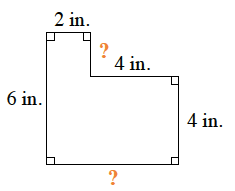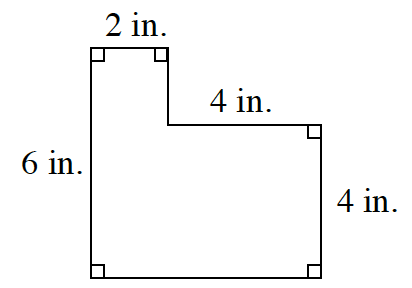### Home > MC2 > Chapter 3 > Lesson 3.1.2 > Problem3-19

3-19.

Find the perimeter of the figure at right.

Remember, perimeter is the total length of all the sides of a shape.

What are the missing lengths for the sides of the shape? Try to fill these in and sum up each side length to get the perimeter.$2+2+4+4+6+6=24$ in.